Significant Findings

Gauss's Law of Gravity applied to Solar Plasma Limb

 Burning Question Why are the Einstein Rings not seen in the star-filled skies?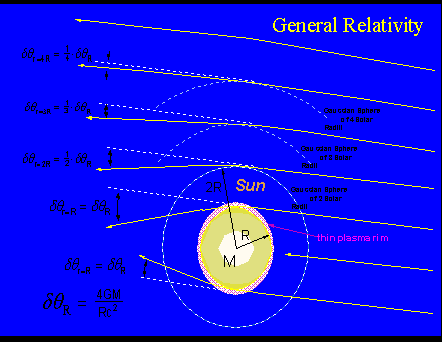Fig 4C Solar light bending as function of the impact parameter R {at solar plasma limb}, 2R, 3R, 4R, etc, "The Observations" versus "General Relativity" explainable with least-time or minimum-energy waves in a plasma exposed to solar gravitational gradient field an assumption of space-time effects or curvature of space to explain gravitational light bending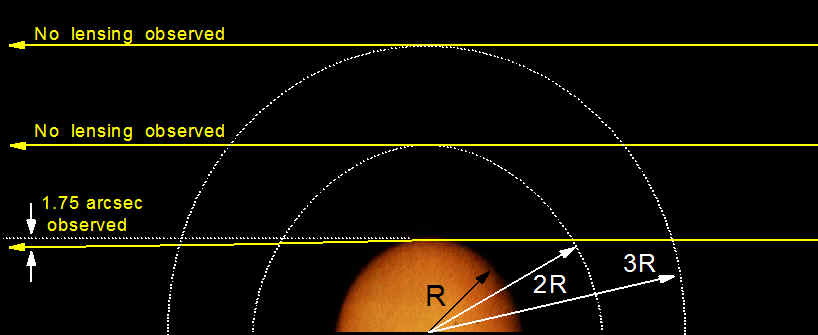Fig 4D: Solar Light Bending according to a Century of Observations

Gravitational light bending, as predicted by General Relativity, should be easily noticeable at multiple solar radii well into the empty vacuum space above the solar plasma rim as depicted in Figure 4D, at impact parameters of 2R, 3R, . . , nR, where R = 695,500 km is one solar radius, M is the solar mass enclosed whose center of mass is concentric to the Gaussian spheres. Since the light bending rule of General Relativity is essentially a 1/R effect, modern technical means in Astrophysics should permit us to easily detect a light bending effect at the impact parameters listed.

 Gravitational Deflection as function of Impact Parameter (in units of the solar radius R) Impact Parameter (solar radii R) Observational History (arsec) General Relativity (arcsec) R 1.75 1.75 2R < 0.875 to negligible 1/2 of 1.75 3R negligible 1/3 of 1.75 nR not observed 1/n of 1.75

 Remarkably as it may seem, however, historically the solar light bending effect has been observed only at the solar rim, the refractive plasma atmosphere of the sun. This is strongly confirmed by a large number of very-long-baseline-interferometer (VLBI) measurements on the gravitational deflection of microwaves from radio pulsar sources that were deflected at the thin plasma rim of the sun at precisely the angle of 1.75 arcsec. See the illustration in Figure 10. The observed solar grazing effect of rays of star light at the thin plasma limb (a thickness that is greatly exaggerated here) is depicted in the following animated illustration:Fig 5  Animated Image is taken from http://science.nasa.gov/science-news/science-at-nasa/2004/26mar_einstein/

 It is interesting to note that the focal length F of  the sun of radius R is determined to be roughly 550 astronomical units (AU's), nearly 14 times the mean orbital radius of Pluto, according to the equation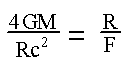The focal length F and the radius R of the sun are expressed in the same units. As depicted in Figure 7, the light rays from a distant star will come to a focus at a distance of roughly 550 AU's, assuming a convergence angle of 1.75 arcsec. It has been a long desire of many astrophysicists, to include SETI study groups and the International Academy of Astronautics, to send spacecrafts loaded with observational instruments 550 AU's out to the focal point of the solar plasma lens.

The Focal Length of the Plasma Limb of a Sun-like Lensing Star

{assume all light rays are coming from sources at infinite distances}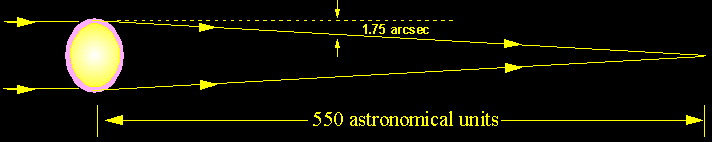observed at plasma focus

Fig 6  Einstein Ring as would be seen at Plasma Focus of the Sun

Any sun-like star of 1 MSUN and 1 RSUN will deflect starlight at 1.75 arcsec at the minimum impact parameter corresponding to the thin plasma limb of that star. The stellar plasma limb of any such lensing star will deflect light of a distant stellar light source. The lensed light rays will come to a focus at a distance of approximately 550 AU's from the lens. Enormous observational evidence supports an  "indirect" interaction (not a "direct" interaction) between the gravitational gradient field of the lensing sun or stars acting on the plasma limbs which in tern acts on the rays of starlight; thus, an "indirect interaction" between the gravitational gradient field of the lensing star and the light rays of distant stars. The visibility of Einstein Rings produced by deflected light rays at impact parameters corresponding to the plasma limbs of stars is consistent with all observations.

Gravitational Deflection as function of Impact Parameter

Supported by History of Observational Evidence

{assume all light rays are coming from sources at infinite distances}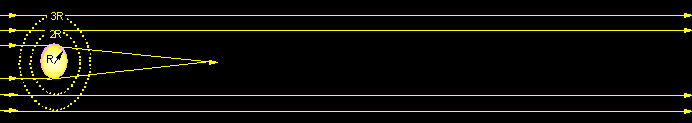High Impact ParameterEinstein Rings NOT OBSERVED

Fig 7  GRAVITATIONAL DEFLECTION as function of IMPACT PARAMETER (The Evidence)

 At the plasma limb of the sun and the stars, historically, the gravitational deflection of light and microwaves are clearly observed. At high impact parameters significantly greater than 1 solar radius and 1 stellar radius, i.e., in the plasma-free vacuum space above the sun and stars, the observed bending of stellar light rays are found to severely deviate from the light bending rule of General Relativity. In the star-filled skies, Einstein Rings produced by high impact parameter light rays are yet to be observed. In this region in the plasma-free vacuum space, the bending of stellar light rays are yet to be observed.

The Observations

The gravitational light bending effect of 1.75 arcsec is constantly being observed at the solar plasma limb and is predicted to come to a focus at 550 AU's. It is predicted by a pure classical least-time / minimum-energy calculation which is independent of the assumptions of General Relativity. The plasma-limb deflection of 1.75 arcsec has been confirmed by a host of VLBI measurements on microwaves emitted from extra galactic quasar radio sources.

At the analytical Gaussian sphere of 2 solar radii the rays of starlight is predicted by General Relativity to be deflected at an angle of 0.875 arcsec which will come to a focus at a factor of 4 times 550 AU's or 2200 AU's. At this impact parameter, there is poor agreement with General Relativity for both starlight and microwaves.

At the analytical Gaussian sphere of 3 solar radii General Relativity predicts a deflection of 0.583 arcsec which will come to a focus at a factor of 9 times 550 AU's or at a focal length of 4950 AU's. At this impact parameter and beyond there is very poor to no agreement at all with General Relativity.

A clear fact supported by observational evidence: An Einstein Ring produced by light rays passing at high impact parameters, i.e., passing clearly above the lensing star in a plasma-free vacuum space, i.e., at impact parameters of multiple stellar radii is yet to be observed. A sun-like lensing star of 1 solar mass and 1 solar radius will deflect rays of starlight at 1.75 arcsec which will come to a focus at approximately 550 AU's.

Gravitational Deflection Predicted by General Relativity

{assume all light rays are coming from sources at infinite distances}NO EVIDENCE

for

Einstein Rings

from high impact parameter rays

Fig 8  Gravitational Deflection Predicted by General Relativity

A minimum-energy calculation shows that electromagnetic waves propagating in a plasma atmosphere exposed to the gravitational gradient field of sun will deflect precisely at the angle of 1.75 arcsec at an impact parameter corresponding to the plasma limb of the sun. This was accomplished from pure classical assumptions of a minimum-energy or least-time path of electromagnetic waves propagating in a plasma atmosphere exposed to the gravitational gradient field of sun. The results was published for the very first time in the book "Discourses & Mathematical Illustrations pertaining to the Extinction Shift Principle under the Electrodynamics of Galilean Transformations".  The least-time calculation has been confirmed by a host of long-base-interferometer measurements of microwaves from radio pulsar sources that were deflected at the thin plasma rim of the sun at precisely the angle of 1.75 arcsec. (For details on this subject matter see Topic 10 "PUBLICATIONS" under Menu of Significant Findings).

As already stated, a history of VLBI measurements have recorded a plasma-limb deflection of microwaves at exactly 1.75 arcsec of microwaves emitted from extra galactic quasar radio sources. At astronomical distances the rays of microwaves are virtually parallel with one another as can be detected by modern astronomical instruments as depicted in Figure 9. As the VLBI detectors involving microwave antennas stationed on Earth based observatories move along an Earth orbit, the gravitational deflection of the rays of microwaves are recorded by researchers conducting these experiments. Historically, the rays of microwaves are observed to deflect only at the angle of 1.75 arcsec, which corresponds to an impact parameter of exact one (1) solar radii or R = 695,500 km. Only at this impact parameter will any star of one solar mass and one solar radius theoretically deflect a ray of microwaves according to the light bending rule of General Relativity at the angle of 1.75 arcsec. If the light bending rule of General Relativity were actually valid then a gravitational deflection of microwaves should also be observable at other impact parameters, namely, at the impact parameter of 2R to reveal a gravitational deflection of exactly 0.875 arcsec, at 3R to reveal a gravitational deflection of exactly 1/3 of 1.75 arcsec, etc., etc. Historically, deflections of microwaves are yet to be recorded at impact parameters greater than that of the solar radius R to observe deflections at angles less than that of 1.75 arcsec.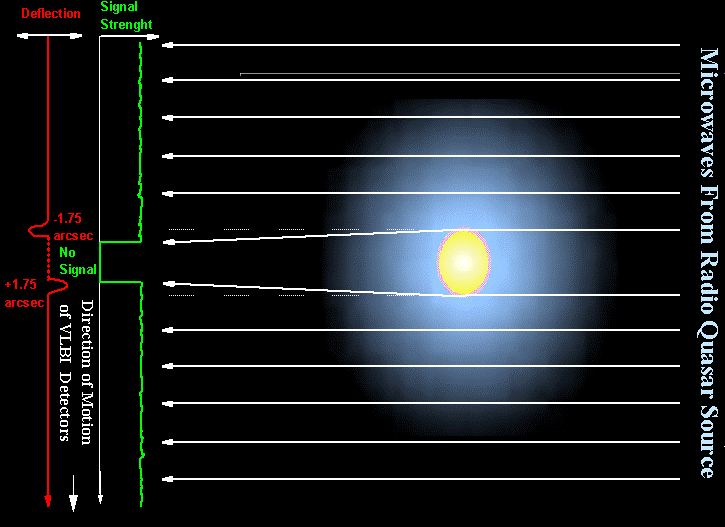Fig 9 Microwaves from Radio Quasar Sources deflected only at the Solar Plasma Limb observed to deflect only at the angle of exactly 1.75 arcsec only at the impact parameter of one (1) solar radius R

Also assuming the validity of the light bending rules of General Relativity, the rays of starlight at high impact parameters relative to the lensing stars should produce Einstein rings all over the night skies whose diameters are considerably larger than the apparent disc size of the lensing stars. There are an infinite number of light rays that could theoretically form an Einstein ring given the geometry of potential lensing stars and the position of an Earth based Einstein ring observer. The images of such Einstein rings in the star-filled night skys should be easily detectable using modern astronomical means.  These Einstein rings are yet to be observed anywhere in the star-filled skies using modern technical means.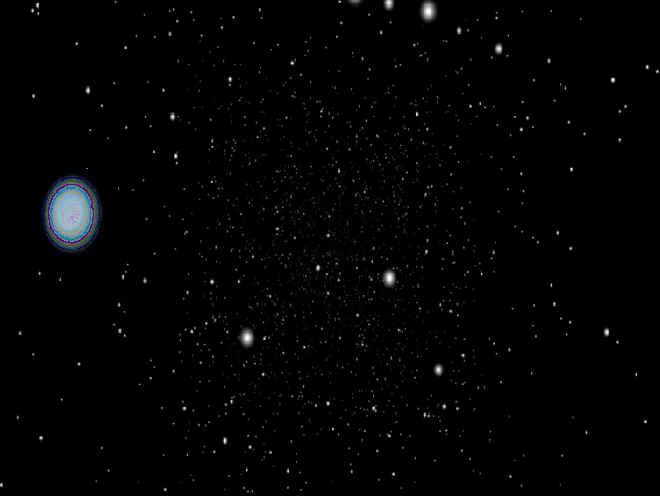Fig 10

Astrophysical evidence confirms that the rays of starlight are lensed only at the plasma limbs of stars. If the light bending rule of General Relativity were indeed valid, which suggests a direct interaction between gravitation and electromagnetism, then all Astrophysical observations of deep space would be totally denied to all modern Astronomy. The deep space images and the Astrophysics thereof would be completely blurred and filled with Einstein rings and arcs.

 Burning Question that's no longer Burning Where are the Einstein Rings among the star-filled skies?